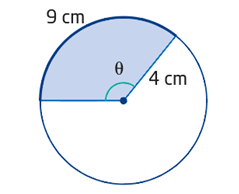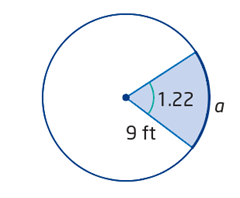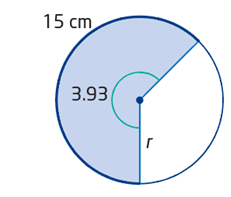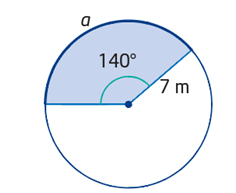# Radian measure and arc length

### Radian measure and arc length

#### Lessons

$arc\;length= r \cdot \theta\;radian$
• 1.
Use the information in each diagram to determine the value of the variable

a)b)c)d)• 2.
A 20 cm long pendulum swings through an arc length of 60 cm. Through
what angle, in both degrees and radians, does the pendulum swing?